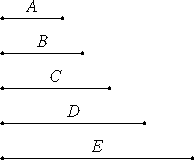Proposition 11

If as many numbers as we please beginning from a unit are in continued proportion, then the less measures the greater according to some one of the numbers which appear among the proportional numbers.

Let there be as many numbers as we please, B, C, D, and E, beginning from the unit A and in continued proportion.I say that B, the least of the numbers B, C, D, and E, measures E according to one of the numbers C or D.

VII.15

Since the unit A is to B as D is to E, therefore the unit A measures the number B the same number of times as D measures E. Therefore, alternately, the unit A measures D the same number of times as B measures E.

But the unit A measures D according to the units in it, therefore B also measures E according to the units in D, so that B the less measures E the greater according to some number of those which have place among the proportional numbers.

Therefore, if as many numbers as we please beginning from a unit are in continued proportion, then the less measures the greater according to some one of the numbers which appear among the proportional numbers.

Q.E.D.

Corollary

And it is clear that, whatever place the measuring number has, reckoned from the unit, the same place also has the number according to which it measures, reckoned from the number measured, in the direction of the number before it.

Guide

This proposition, along with the comment made in the corollary, says that ak divides an the number an-k times.

Use of this proposition

The corollary is used in the next proposition while the proposition itself is used in the one following that.